# RBI Assistant Pre 2022 Quantitative Aptitude Quiz – 12

## RBI Assistant 2022 Quantitative Aptitude Quiz

Quantitative aptitude is a quite important section of various banking exams. Many candidates face difficulty in solving Quantitative Aptitude Questions. Even if the portion is difficult, if you practice enough questions and learn the basic concepts, you can surely do well. So, here we bring to you the RBI Assistant 2022 Quantitative Aptitude Quiz. Candidates will be provided with a detailed explanation of each question in this RBI Assistant 2022 Quantitative Aptitude Quiz. This RBI Assistant 2022 Quantitative Aptitude Quiz is completely FREE for the Candidates. This RBI Assistant 2022 Quantitative Aptitude Quiz contains various questions ranging from simple to difficult levels. This RBI Assistant 2022 Quantitative Aptitude Quiz will assist the candidates with upgrading their preparation for upcoming examinations.

Directions (1-5): In each of these questions, two equations (I) and (II) are given. You have to solve both the equations and give answer.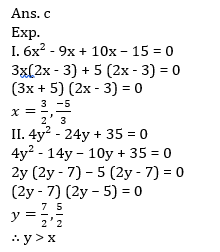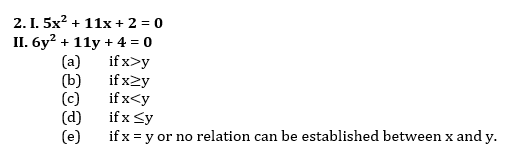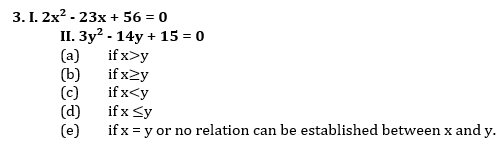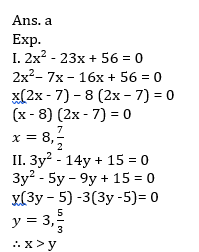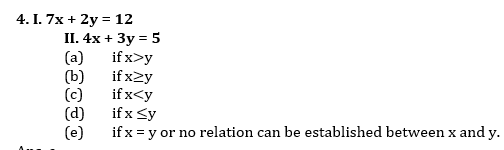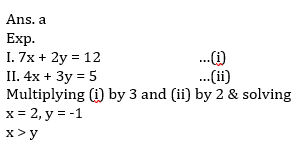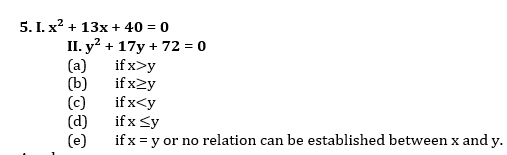6. A boat travels in upstream. If the speed of boat in upstream in decreased by 40% then it is equal to the speed of current and speed of boat in still water is given as 240 km/hr. Then find upstream speed of boat? (in km/hr).
(a) 120
(b) 180
(c) 150
(d) 210
(e) 125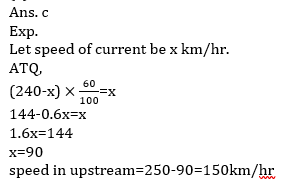7. The age of father is 4 times the age of his son. 5 years ago the age of son was 1/5 times of his father age. Find the present age of son.
(a) 20 yrs
(b) 30 yrs
(c) 28 yrs
(d) 25 yrs
(e) 32 yrs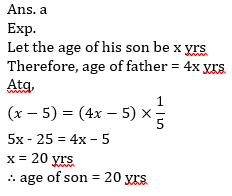8. Anurag invested some amount for 3 yrs at rate of 16 2/3% per annum at CI. The difference of CI obtained only on 3rd year and C.I. obtained only on second year is Rs 210. Find the amount invested by Anurag?
(a) Rs 6,220
(b) Rs 6,480
(c) Rs 8,420
(d) Rs 7,420
(e) Rs 8,240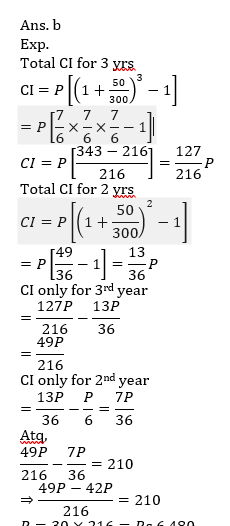9. Perimeter of rectangle is equal to the perimeter of square whose area is 400 cm² and length of rectangle is 40% more than the side of a square then find the area of rectangle?
(a) 248 cm²
(b) 420 cm²
(c) 356 cm²
(d) 336 cm²
(e) 348 cm²10. A question is given to Saket, Ankush and Prem. Probability that Saket, Ankush and Prem can solve the question is 1/3, 2/5 and 1/2. Find the probability that the question will be solved?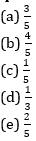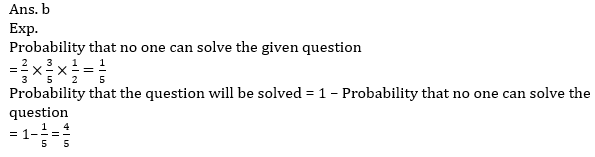#### Attempt Quantitative Aptitude Topic Wise Online Test Series

RBI Assistant Prelims Online Test Series 2022

Recommended PDF’s for:

#### Most important PDF’s for Bank, SSC, Railway and Other Government Exam : Download PDF Now

AATMA-NIRBHAR Series- Static GK/Awareness Practice Ebook PDF Get PDF here
The Banking Awareness 500 MCQs E-book| Bilingual (Hindi + English) Get PDF here
AATMA-NIRBHAR Series- Banking Awareness Practice Ebook PDF Get PDF here
Computer Awareness Capsule 2.O Get PDF here
AATMA-NIRBHAR Series Quantitative Aptitude Topic-Wise PDF Get PDF here
AATMA-NIRBHAR Series Reasoning Topic-Wise PDF Get PDF Here
Memory Based Puzzle E-book | 2016-19 Exams Covered Get PDF here
Caselet Data Interpretation 200 Questions Get PDF here
Puzzle & Seating Arrangement E-Book for BANK PO MAINS (Vol-1) Get PDF here
ARITHMETIC DATA INTERPRETATION 2.O E-book Get PDF here
3